# ML-Lecture 3.2

``````%load_ext autoreload
%matplotlib inline
import sys

# or wherever you have saved the repo
sys.path.append('/Users/tlee010/Desktop/github_repos/fastai/')

from fastai.imports import *
from fastai.structured import *
from pandas_summary import DataFrameSummary
from sklearn.ensemble import RandomForestRegressor, RandomForestClassifier
from IPython.display import display
from sklearn import metrics
``````

### Load in data from last lesson - from feather

``````import feather
print('import complete')
``````
``````import complete
``````

### Load in our data from last lesson

``````# loading the data (large)
# %time df_raw = pd.read_csv('/Users/tlee010/kaggle/bulldozers/Train.csv', low_memory=False, parse_dates=["saledate"])
``````
``````set_plot_sizes(12,14,16)
``````
``````??set_plot_sizes
``````
``````def set_plot_sizes(sml, med, big):
plt.rc('font', size=sml)          # controls default text sizes
plt.rc('axes', titlesize=sml)     # fontsize of the axes title
plt.rc('axes', labelsize=med)    # fontsize of the x and y labels
plt.rc('xtick', labelsize=sml)    # fontsize of the tick labels
plt.rc('ytick', labelsize=sml)    # fontsize of the tick labels
plt.rc('legend', fontsize=sml)    # legend fontsize
plt.rc('figure', titlesize=big)  # fontsize of the figure title
File:      ~/Desktop/github_repos/fastai/fastai/structured.py
``````

### Break the data from last lesson into Train and Test

``````df_trn, y_trn, _ = proc_df(df_raw, 'SalePrice')

def split_vals(a,n): return a[:n], a[n:]
n_valid = 12000
n_trn = len(df_trn)-n_valid
X_train, X_valid = split_vals(df_trn, n_trn)
y_train, y_valid = split_vals(y_trn, n_trn)
raw_train, raw_valid = split_vals(df_raw, n_trn)
``````

• How look at the machine learning models to gain insight or learn about the data.
• How to look at larger datasets

#### Q: Is there any intuition on when to apply the random forest model?

• A: the answer is rephrase “in what situation would i try other models” - unstructured data, images, sound files, then try deep learning. Similarly special cases may align better with Collaborative filtering which is a different model.

## Large Dataset Considerations - Example Grocery Kaggle

This is what we call a relational dataset. This is what we call a start schema. It’s called a star schema, because it is in the middle with other tables connecting to it (outside). There’s also a snowflake design which has additional branches on the edges.

There’s a central transactions dataset. In this case it tracks what was sold, who bought it, and what they bought.

In this case, when we run it, the file is HUGE 5GB.

We will use `%time` and `%%time` in the jupyter notebook blocks to time some of the longer processes

#### Rule 1: don’t load in the entire dataset the first time. Use Shuf to start and sample

• Use pandas to help decide the datatypes needed

#### Set the Path

``````path = '/Users/tlee010/kaggle/grocery/train.csv'
``````
`````` types = {'id':'int64',
'item_nbr':'int32',
'store_nbr':'float32',
'onpromotion':'object',
}
``````
``````%%time
import pandas as pd
df_all = pd.read_csv(path, parse_dates= ['date'], dtype = types, infer_datetime_format=True)
``````
``````CPU times: user 2min 26s, sys: 21.6 s, total: 2min 47s
Wall time: 2min 50s
``````

# Removed Grocery Kaggle code + notes due to active competition

### Random Forest

Can only do binary divisions. It’s ability to understand locations and other meta data is limited. There’s a kaggle kernel, take the last two weeks, take the average sales by date, item number and on promotion submit. If you submit that you get 30th.

Your job is how do you start with that model and make it a little bit better?

• How can you make some additional features?
• The coding you do for machine learning is really frustrating

1st place

3rd place

## 1. Random Forests

``````import feather
print('import complete')
``````
``````import complete
``````
``````df_trn, y_trn, _ = proc_df(df_raw, 'SalePrice')
``````
``````def split_vals(a,n): return a[:n], a[n:]
n_valid = 12000
n_trn = len(df_trn)-n_valid
X_train, X_valid = split_vals(df_trn, n_trn)
y_train, y_valid = split_vals(y_trn, n_trn)
raw_train, raw_valid = split_vals(df_raw, n_trn)
``````
``````def rmse(x,y): return math.sqrt(((x-y)**2).mean())

def print_score(m):
res = [rmse(m.predict(X_train), y_train), rmse(m.predict(X_valid), y_valid),
m.score(X_train, y_train), m.score(X_valid, y_valid)]
if hasattr(m, 'oob_score_'): res.append(m.oob_score_)
print(res)
``````
``````df_raw.head(3)
``````
.dataframe thead tr:only-child th { text-align: right; }
``````.dataframe thead th {
text-align: left;
}

.dataframe tbody tr th {
vertical-align: top;
}
``````
SalesID SalePrice MachineID ModelID datasource auctioneerID YearMade MachineHoursCurrentMeter UsageBand fiModelDesc ... saleDay saleDayofweek saleDayofyear saleIs_month_end saleIs_month_start saleIs_quarter_end saleIs_quarter_start saleIs_year_end saleIs_year_start saleElapsed
0 1139246 11.097410 999089 3157 121 3.0 2004 68.0 Low 521D ... 16 3 320 False False False False False False 1163635200
1 1139248 10.950807 117657 77 121 3.0 1996 4640.0 Low 950FII ... 26 4 86 False False False False False False 1080259200
2 1139249 9.210340 434808 7009 121 3.0 2001 2838.0 High 226 ... 26 3 57 False False False False False False 1077753600

3 rows × 65 columns

#### Mean vs. Std. for predictions

We already know how to get predictions. We take the average value in each leaf node to get the prediction. But normally we dont want just a prediction we also want an interval.

If we have seen that many rows much like a new data set. Then we won’t have path through the tree for a specifically new row, it will end up in weird places.

What if instead of mean, we took the standard deviation per node. If its a common row, the tree will learn to make a good predictions. The standard deviations of the predictions of the trees. Gives us relative understanding of how confident we are of this prediction.

That is not something that exists in scikit learn. So we will create it.

# Confidence based on tree variance

We don’t need a massively large dataset for random forest. We just need enough to have consistent examples that gives an indicator if we are on the right track.

``````set_rf_samples(50000)
``````

## 40 Estimators (Trees)

``````m = RandomForestRegressor(n_estimators=40, min_samples_leaf=3, max_features=0.5, n_jobs=-1, oob_score=True)
m.fit(X_train, y_train)
print_score(m)
``````
``````[0.20586503432923145, 0.24932815554452017, 0.91142727542837831, 0.8889827098012647, 0.89436793611776322]
``````

#### From last time, go through each tree and stack predictions.

Then we show how to calculate the mean and how to calculate the std.

``````%time preds = np.stack([t.predict(X_valid) for t in m.estimators_])
np.mean(preds[:,0]), np.std(preds[:,0])
``````
``````CPU times: user 1.33 s, sys: 8.62 ms, total: 1.34 s
Wall time: 1.34 s

(9.2300841617140215, 0.26126856453842179)
``````

#### List comprehensions - runs single thread (doesn’t take advantage of multi-core processes

fastai provides a handy function called parallel trees that uses multi threaded support from sklearn

``````??parallel_trees
``````
``````def parallel_trees(m, fn, n_jobs=8):
return list(ProcessPoolExecutor(n_jobs).map(fn, m.estimators_))
``````
``````def get_preds(t): return t.predict(X_valid)
%time preds = np.stack(parallel_trees(m, get_preds))

# mean , std
np.mean(preds[:,0]), np.std(preds[:,0])
``````
``````CPU times: user 66.7 ms, sys: 72 ms, total: 139 ms
Wall time: 430 ms

(9.2300841617140215, 0.26126856453842179)
``````

### Ref: Pandas Plotting

https://pandas.pydata.org/pandas-docs/stable/visualization.html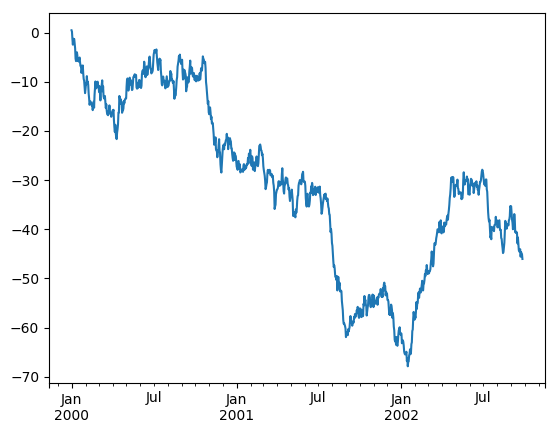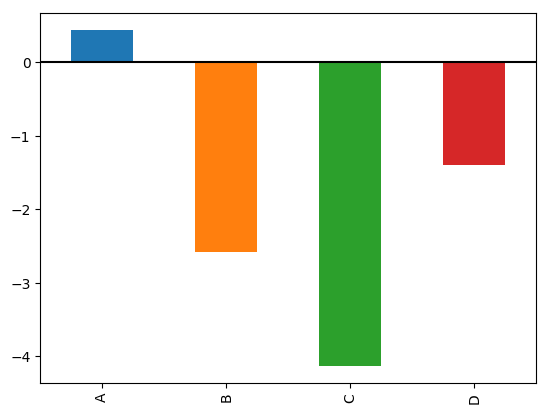#### Looking at Enclosure as an important feature

``````x = raw_valid.copy()

# standard dev
x['pred_std'] = np.std(preds, axis=0)

# store the mean
x['pred'] = np.mean(preds, axis=0)
x.Enclosure.value_counts().plot.barh();
``````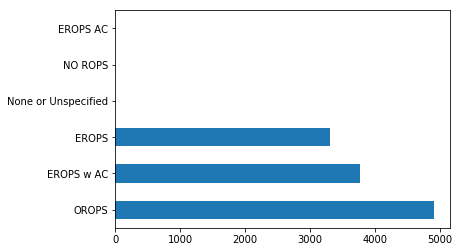#### Let’s group by each type of Enclosure and see what the prediction

``````flds = ['Enclosure', 'SalePrice', 'pred', 'pred_std']
enc_summ = x[flds].groupby('Enclosure', as_index=False).mean()
enc_summ
``````
.dataframe thead tr:only-child th { text-align: right; }
``````.dataframe thead th {
text-align: left;
}

.dataframe tbody tr th {
vertical-align: top;
}
``````
Enclosure SalePrice pred pred_std
0 EROPS 9.849178 9.840166 0.277068
1 EROPS AC NaN NaN NaN
2 EROPS w AC 10.623971 10.579176 0.266230
3 NO ROPS NaN NaN NaN
4 None or Unspecified NaN NaN NaN
5 OROPS 9.682064 9.683984 0.224335
``````enc_summ = enc_summ[~pd.isnull(enc_summ.SalePrice)]
enc_summ.plot('Enclosure', 'SalePrice', 'barh', xlim=(0,11));
``````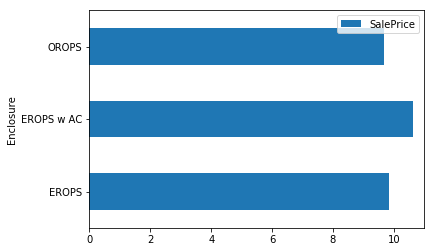#### Add Error bars. Will tell us if we are not confident

``````enc_summ.plot('Enclosure', 'pred', 'barh', xerr='pred_std', alpha=0.6, xlim=(0,11));
``````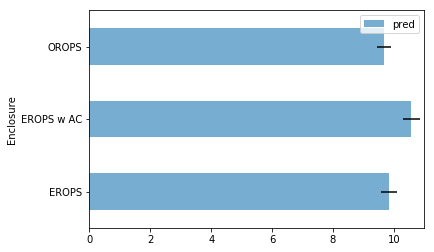#### Add to Product size - consider

``````raw_valid.ProductSize.value_counts().plot.barh();
``````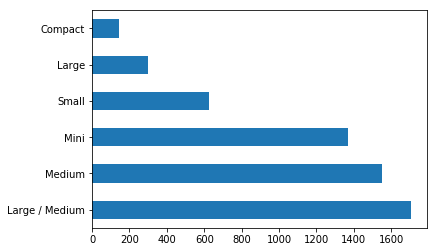We see that we have some big intervals for compact and for large and if we look at the chart above, its probably because the dataset size is too small.

``````flds = ['ProductSize', 'SalePrice', 'pred', 'pred_std']
summ = x[flds].groupby(flds).mean()
summ
``````
.dataframe thead tr:only-child th { text-align: right; }
``````.dataframe thead th {
text-align: left;
}

.dataframe tbody tr th {
vertical-align: top;
}
``````
SalePrice pred pred_std
ProductSize
Compact 9.735093 9.849076 0.334910
Large 10.470589 10.389998 0.359176
Large / Medium 10.691871 10.658287 0.299063
Medium 10.681511 10.627427 0.287277
Mini 9.535147 9.562571 0.251085
Small 10.324448 10.318753 0.318815

### Look at a ratio std vs. the actual prediction

``````(summ.pred_std/summ.pred).sort_values(ascending=False)
``````
``````ProductSize
Large             0.034569
Compact           0.034004
Small             0.030897
Large / Medium    0.028059
Medium            0.027032
Mini              0.026257
dtype: float64
``````

### Feature Importance

It’s not normally enough to just to know that a model can make accurate predictions - we also want to know how it’s making predictions. The most important way to see this is with feature importance.

``````fi = rf_feat_importance(m, df_trn); fi[:10]
``````
.dataframe thead tr:only-child th { text-align: right; }
``````.dataframe thead th {
text-align: left;
}

.dataframe tbody tr th {
vertical-align: top;
}
``````
cols imp
37 Coupler_System 0.095873
13 ProductSize 0.089217
14 fiProductClassDesc 0.082386
39 Hydraulics_Flow 0.059927
2 ModelID 0.059114
63 saleElapsed 0.050549
10 fiSecondaryDesc 0.043213
38 Grouser_Tracks 0.040982
19 Enclosure 0.040818
``````??rf_feat_importance
``````

This function is a simple wrapper around the standard SKLEARN model objects. Most of the classifications have `.feature_importance_` as a standard attribute of the model objects

``````def rf_feat_importance(m, df):
return pd.DataFrame({'cols':df.columns, 'imp':m.feature_importances_}
).sort_values('imp', ascending=False)
``````

#### Plot the distribution of importance across different features

``````fi.plot('cols', 'imp', figsize=(10,6), legend=False);
``````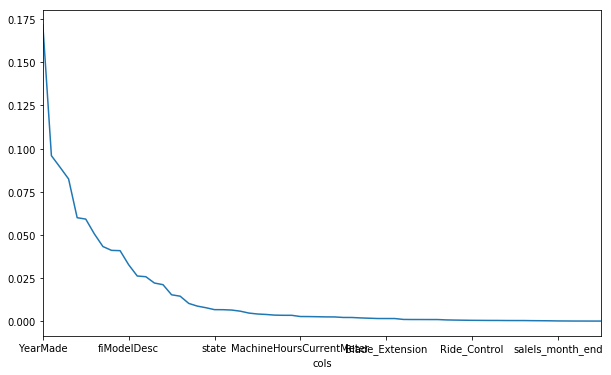``````def plot_fi(fi): return fi.plot('cols', 'imp', 'barh', figsize=(12,7), legend=False)
``````
``````plot_fi(fi[:30]);
``````

### How to interpret?

1. Review data w/ experts: Sit with the client and the data dictionary and go through each of the top features.

2. **If client says ‘that makes no sense’ **: doesn’t mean anything. It may just mean that there’s a miscommunication in the data. Either in what was provided, or even all the way back to when it was recorded. Sometimes the default value may be misleading.

3. data leakage : that back at time t1, they wouldn’t have all the data that we have looking back.

### What next?

• try throwing some data away and see if that matters

#### Keep only ‘important’ features and make a smaller dataframe

``````to_keep = fi[fi.imp>0.005].cols; len(to_keep)
``````
``````24
``````

#### Re-run the model to see how stable the feature importance is

``````df_keep = df_trn[to_keep].copy()
X_train, X_valid = split_vals(df_keep, n_trn)
``````
``````m = RandomForestRegressor(n_estimators=40, min_samples_leaf=3, max_features=0.5,
n_jobs=-1, oob_score=True)
m.fit(X_train, y_train)
print_score(m)
``````
``````[0.20690392074364916, 0.24477691611214772, 0.9105310651527877, 0.89299873999387613, 0.89417513895254686]
``````
``````fi = rf_feat_importance(m, df_keep)
plot_fi(fi);
``````

### Interpretation

When you limited top importance. You may have removed collinearity, then you may be consolidated some of the feature importance and ‘clear up’ the feature importance (have a clear picture).

• We see year made now looks much much more important than in the previous graph

### How can you verify the feature importance? Decoupled Shuffling

Take our dataset that we are trying to predict which is price. We have all the independent variables. In this case we had 25 different variables.

How do we figure out how important `year_made` is?

1. run 25 baseline We run the model of the top25, and consider it baseline. Keep the model the same, then re-run predict with the following steps.
2. Shuffle within col Lets take the `year made` column and randomly shuffle (vertically, and decouple from rows)
3. Compare scores Compare the scores between the baseline + re-shuffled version
4. Shuffle other cols and note change in score Do the same with the other top features and note the decrease in score.
``````
``````
3 Likes

Just to clarify - the ‘shuffling’ approach you discuss right at the end isn’t a way to verify feature importance; it is how sklearn does variable importance. It’s a description of the algorithm that sklearn uses behind the scenes.

2 Likes